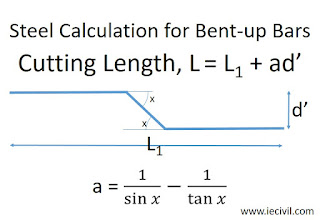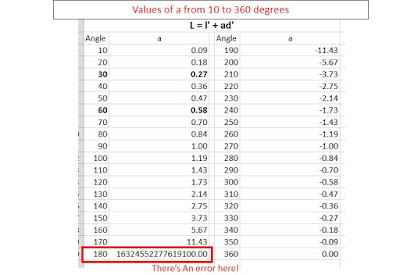# ieCivil

Engineering the Digital Civilization

## Saturday, 28 July 2018

Steel quantity calculations is a complex task and can be automated to a great extent by using formulas and innovative solutions.

While I was a student of engineering, in the final year we had this subject called as QSCT which included Estimation of quantities. A part of the subject included the calculation of steel quantities. Generally, reinforcement steel is calculated in cutting length and then later this length is converted into weight by multiplying with the factor.

Generally, Bent-ups are of 3 types viz. 30, 45 and 60 degrees. The corresponding additions to the bent angles are 0.26d, 0.42 and 0.57d respectively, remembering these values is an absolute pain and it is also a bit tricky to code for these. I prefer learning how stuff come into existence like the complete derivation of the factors. So now in order to remember and recall these factors during exams, I came up with a simple formulaThe formula for Calculation of Bent-up Bar Lenght

Then just by changing the angle, you can get the length that we're supposed to add in the cutting length of the steel. I just remembered the formula and later using the calculator I used to compute the factors. Also while coding a spreadsheet stuffs become extremely simple and easy to handle. This is how I implement the above formula in Mircosoft Excel or any other spreadsheet software.Implementation of Formula in Excel
Now once you've learned this you can simply automate much of your tasks in the computation and make it simple and time-saving.

Just for fun purposes I made computed the value of a for angles ranging from 0 to 360 degrees. As it is It was just pulled and drag stuff so it didn't take much of the time.Values of a from 10 to 360 degrees
From the above table, it can be noted that the formula gives an error at 180 degree but the error isn't relevant. I think it happens because 180 degrees means that the bars are out of phase but collinear to the cover spacing. I would like to know & learn about the scientific reason for that error.